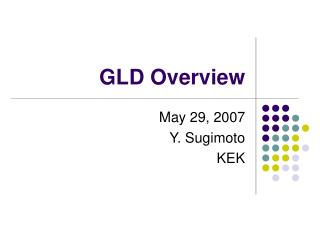DownloadDownload PresentationGLD Overview

# GLD Overview

Télécharger la présentation## GLD Overview

- - - - - - - - - - - - - - - - - - - - - - - - - - - E N D - - - - - - - - - - - - - - - - - - - - - - - - - - -
##### Presentation Transcript

1. GLD Overview May 29, 2007 Y. Sugimoto KEK

2. Baseline Design • Large gaseous central tracker; TPC • Large-radius, high-granularity ECAL with W/Scinti sandwich structure • Large-radius, medium granularity, thick (~6l) HCAL with Pb(Fe)/Scinti. sandwich structure • Forward CAL (FCAL and BCAL) down to 5mrad • Precision Si micro-vertex detector • Si inner tracker (barrel and forward) • Si endcap tracker • Beam profile monitor in front of BCAL • Muon detector interleaved with iron plates of the return yoke • Moderate magnetic field of 3T

3. Baseline Design Return yoke design modified from DOD to reduce the total size of the detector and exp-hall size

4. Baseline Design

5. Baseline Design Back-scattering from BCAL should not hit TPC directly

6. Detector Parameters • VTX • 6 layers (3 doublets) • R=20(18) mm – 50 mm • SIT • 4 layers • R=9 cm – 30 cm • Bunch ID capability • TPC • R=45 cm – 200 cm • Z=230 cm • MUO • 8/10 layers interleaved with 25-30 cm thick iron slab of return yoke

7. Detector Parameters • CAL/PFA

8. Why is GLD large? • How much iron do we need? • B-field calculation based on a toy model using a FEA program was done • BR2, tECAL, tHCAL, G1, G2; fixed • Leakage field at Z=10 m was estimated as a function of B, and tFe • tFe to satisfy the leakage limit of 100G was obtained for each B

9. Leakage B-field • GLD-like • BR2=13.23 • tECAL=0.17m • tHCAL=1.23m • G1=G2=0.5m BZ=10m Leakage limit Andrei put the limit to 50G, but 100G can be reduced to <50G by low cost Helmholtz coil tFe (m)

10. Leakage B-field • LDC-like • BR2=10.24 • tECAL=0.17m • tHCAL=1.13m • G1=0.46m • G2=0.49m BZ=10m tFe (m)

11. Leakage B-field • SiD-like • BR2=8.06 • tECAL=0.13m • tHCAL=1.09m • G1=0.21m • G2=0.63m BZ=10m tFe (m)

12. B and R GLD-like LDC-like SiD-like R (m) B (T) For a given BR2, larger B (smaller RCAL) gives larger detector size

13. B and Cost • GLD-like detector model • Unit cost assumption • ECAL: 6.8M\$/m3 • HCAL: 0.16M\$/m3 • Fe: 42k\$/m3 • Solenoid: 0.523x[Estore]0.662 M\$ Cost (M\$) B-field dependence of the total cost (CAL+Sol.+Fe) is very small B (T)

14. Summary • GLD is the largest detector among the three PFA detectors • GLD is the largest NOT because it has the largest inner radius of the calorimeter, but because it has the largest BR2, the thickest HCAL, and the smallest leakage field